# Type Casting in C#

## Type Casting in C# with Examples

In this article, I am going to discuss Type Casting in C# with Examples. Please read our previous article, where we discussed Literals in C# with Examples. At the end of this article, you will understand what is Type Casting and why, and when to use Type Casting in C# Application with Examples.

##### What is Type Casting in C#?

In simple words, we can say that Type Casting or Type Conversion in C# is the process to change one data type value into another data type. The Type Conversion is only possible if both the data types are compatible with each other else we will get compile time error saying cannot implicitly convert one type to another type.

According to our business needs, we may need to change the type of data. At the time of the compilation, the C# compiler followed statically-typed i.e., after the declaration of a variable, we cannot declare it again.

Let us understand this with an example. Here, we are creating a variable of int data type. We cannot convert the string value implicitly to the int. Therefore, if we declare the variable “a” as int, we cannot assign the string value Hello to it.

int a;
a = “Hello”; // error CS0029: cannot implicitly convert type string to int

However, we can face a situation when there is a need to copy the value of one variable into another variable or a method parameter of another type. For example, we have a variable integer, and we need to pass it to a method parameter whose type is double.

So, the process of converting the value of one data type (int, float, double, etc.) to another data type ((int, float, double, etc.)) is known as type conversion or typecasting.

##### Types of Type Casting in C#

The type conversion or the typecasting is done automatically by the compiler or even as a developer we can also do it explicitly and hence type casting in C# is classified into two types. They are as follows:

1. Implicit Type Casting
2. Explicit Type Casting
##### Implicit Conversion or Implicit Type Casting / Automatic Type Conversion in C#

The Implicit Conversion or Implicit Type Casting in C# is automatically done by the compiler and in this case, there will be no data loss. Here, the typecasting or type conversion is done from a smaller data type to a larger data type. This type of type conversion is safe.

In implicit Type Conversion, the compiler will automatically convert one type to another. Generally, in the case of implicit Type Conversion, the smaller data types like int (having less memory size) are automatically converted to larger data types like long (having larger memory size).

Implicit Type Casting happens when:

1. The two data types are compatible.
2. When we assign a value of a smaller data type to a bigger data type.

For example, in C#, the numeric data types like byte, short, int, long, double, float, decimal, etc. are compatible with each other but no automatic conversion is supported from numeric type to char type or Boolean type. Also, char and bool are not compatible with each other. So, before converting, the compiler will first check the type compatibility, and then it decides whether it is good to convert or throw some error.

The following diagram shows the implicit types of conversion that are supported by C#: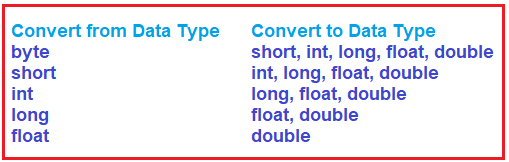##### Example to Understand Implicit Conversion or Implicit Type Casting in C#

Let us understand implicit Type Conversion in C# with an example. In the following example, we have created an integer variable with the name numInt i.e. int numInt = 1500;. Please notice the line, double numDouble = numInt; Here, we are assigning the int type variable value to a double type variable. In this case, the compiler will automatically convert the int type value to double type. This is because both int and double are numeric types and hence the types are compatible. And further, int takes 4-Bytes of memory and double takes 8-Bytes of memory, and hence there is no issue to store 4Bytes of data inside 8-Bytes of memory location. Also, here we have used the GetType() method to check the type of numInt and numDouble variables data type and we are also using sizeof function to check the size of int and double data type.

```using System;
namespace TypeCastingDemo
{
class Program
{
static void Main(string[] args)
{
int numInt = 1500;

//Get type of numInt
Type numIntType = numInt.GetType();

// Implicit Conversion
double numDouble = numInt;

//Get type of numDouble
Type numDoubleType = numDouble.GetType();

// Value Before Conversion
Console.WriteLine(\$"numInt value: {numInt}" );
Console.WriteLine(\$"numInt Type: {numIntType}");
Console.WriteLine(\$"Int Size: {sizeof(int)} Bytes");

// Value After Conversion
Console.WriteLine(\$"numDouble value: {numDouble}");
Console.WriteLine(\$"numDouble Type: {numDoubleType}");
Console.WriteLine(\$"double Size: {sizeof(double)} Bytes");

}
}
}
```
###### Output: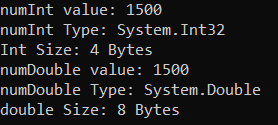Note: In implicit type conversion, the smaller types are converted to larger data types and hence, there is no loss of data during the conversion.

##### Explicit Conversion or Explicit Type Casting in C#

If you want to convert the large data type to a small data type in C#, then you need to do the same explicitly using the cast operator. Explicit Conversion or Explicit Type Casting in C# is done by using the Cast operator. It includes the conversion of larger data types to smaller data types. In the case of Explicit Conversion or Explicit Type Casting. there is a chance of data loss or the conversion might not be succeeded for some reason. This is an unsafe type of conversion.

In Explicit Type Conversion, we explicitly convert one data type to another data type. In this case, the larger data types like double or long (having large memory size) are converted to smaller data types like int, byte, short, float, etc. (having small memory size).

##### Example to Understand Explicit Conversion or Explicit Type Casting in C#

You will get compilation errors when types are not compatible with each other. For example, assigning a double value to an int data type results in a compile-time error as shown in the below example.

```using System;
namespace TypeCastingDemo
{
class Program
{
static void Main(string[] args)
{
double numDouble = 1.23;

// Explicit Type Casting
int numInt = numDouble;

// Value Before Conversion
Console.WriteLine("Original double Value: " + numDouble);

// Value After Conversion
Console.WriteLine("Converted int Value: " + numInt);
}
}
}
```
###### Output: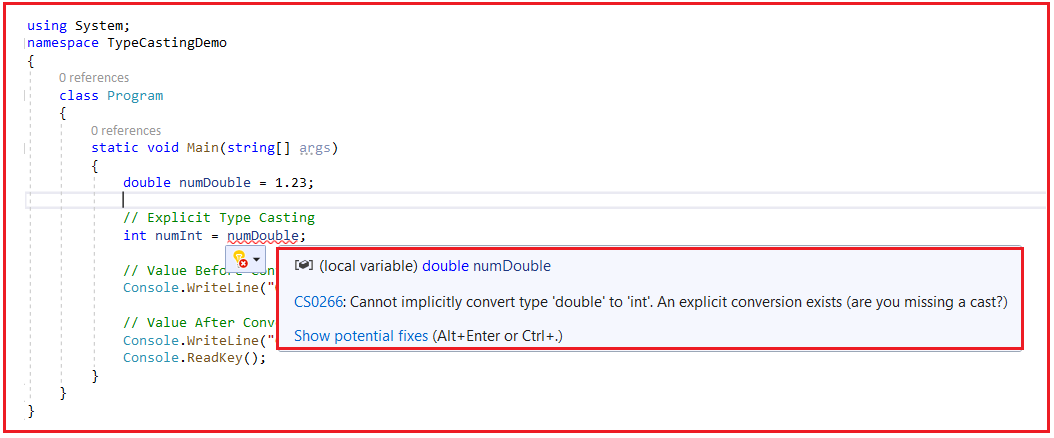As you can see, in the above image, it is giving a compilation error as Cannot implicitly convert type ‘double’ to ‘int’. An explicit conversion exists (are you missing a cast?). It is giving errors as well as it is giving suggestions that are you missing a cast. That means if you want this type of conversion, then you need to explicitly use the cast operator.

So, if we want to assign a value of a larger data type to a smaller data type then you need to perform explicit type casting in C#. This is useful for compatible data types where automatic type conversion is not possible by the compiler. See, whether it is implicit type conversion or explicit type conversion, the type types should be compatible, then only the type conversion is going to be possible.

In the below example, we have created a double variable named numDouble i.e. double numDouble = 1.23;. Notice the lineint numInt = (int)numDouble; Here, (int) is a cast expression that explicitly converts the double type value 1.23 to int type.

```using System;
namespace TypeCastingDemo
{
class Program
{
static void Main(string[] args)
{
double numDouble = 1.23;

// Explicit Type Casting
int numInt = (int)numDouble;

// Value Before Conversion
Console.WriteLine("Original double Value: " + numDouble);

// Value After Conversion
Console.WriteLine("Converted int Value: " + numInt);
}
}
}
```
###### Output: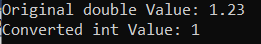Here, you can see that the original value is 1.23 whereas the converted value is 1. That means we have lost some data during the type conversion. This is because we are explicitly converting the larger data type double to smaller type int.

##### Is it always we lose data when we convert a larger type to a smaller type in C#?

The answer is no. It basically depends on the value that we are converting and the size of the data type which is going to store the converted value. For a better understanding, please have a look at the below code.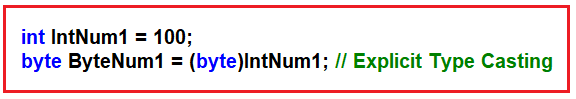In the above case, we will not lose any data. This is because the integer variable holds the value 100 and in byte data type, we can store the values from 0 to 255, and 100 comes under this range and hence no data loss. Now, observe the following code.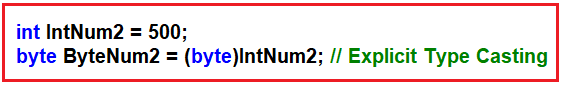In the above case, we will lose the data. This is because the integer variable holds the value 500 and in byte data type, we can store the values from 0 to 255, and 500 does not come under this range, and hence there is data loss. The complete example code is given below.

```using System;
namespace TypeCastingDemo
{
class Program
{
static void Main(string[] args)
{
int IntNum1 = 100;
byte ByteNum1 = (byte)IntNum1; // Explicit Type Casting
// Printing the Original Value and Converted Value
Console.WriteLine(\$"Original Value:{IntNum1} and Converted Value:{ByteNum1}");

int IntNum2 = 500;
byte ByteNum2 = (byte)IntNum2; // Explicit Type Casting
// Printing the Original Value and Converted Value
Console.WriteLine(\$"Original Value:{IntNum2} and Converted Value:{ByteNum2}");
}
}
}
```
###### Output: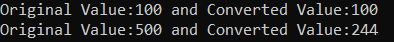As you can see in the above output image, for the first conversion, we don’t have any data loss, but in the second conversion, we have data loss i.e. original value 500 and converted value 244.

##### Conversion with Helper Methods in C#:

Now, please observe the following example. Here, we have a string variable that holds the value 100 and we try to convert that value to an integer type. But this is not possible with the cast operator. Because cast operator will first check the type compatibility and it found that string and int are not compatible with each other because the string is used to store textual data which contains both alphanumeric and int contains only numeric data.

```using System;
namespace TypeCastingDemo
{
class Program
{
static void Main(string[] args)
{
string str= "100";
int i1 = (int)str;

}
}
}
```

When you try to execute the above code, you will get the following compilation error.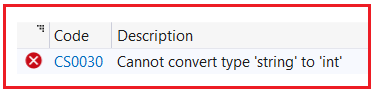So, for the conversion between non-compatible types like integer and string, the .NET Framework provided us with the Convert class, Parse method, and TryParse method. Let us understand these things one by one and see how we can convert non-compatible types in C# with Examples.

##### Convert Class Helper Methods in C#:

The Convert class provides the following methods to convert a value to a particular type. The following methods are going to convert the value irrespective of type compatibility. It means if types are compatible, then it will convert and if types are not compatible, then also it will try to convert.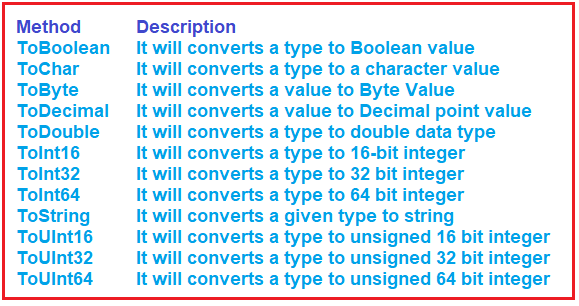For example, if you want to convert a string to an Int type, then you need to use either Convert.ToInt16, or Convert.ToInt32, or Convert.ToInt64. These helper methods are implemented as static methods inside the Convert class and hence you can access them directly. For a better understanding, please have a look at the following example.

```using System;
namespace TypeCastingDemo
{
class Program
{
static void Main(string[] args)
{
string str = "100";
int i1 = Convert.ToInt32(str); //Converting string to Integer

double d = 123.45;
int i2 = Convert.ToInt32(d); //Converting double to Integer

float f = 45.678F;
string str2 = Convert.ToString(f); //Converting float to string

Console.WriteLine(\$"Original value str: {str} and Converted Value i1:{i1}");
Console.WriteLine(\$"Original value d: {d} and Converted Value i2:{i2}");
Console.WriteLine(\$"Original value f: {f} and Converted Value str2:{str2}");
}
}
}
```
###### Output: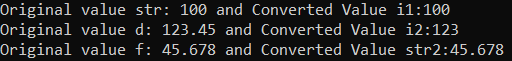When we are using the Convert class helper method to convert a value to a particular type, if types are not compatible, then it will not throw you any error at compile time. At run time, it will try to convert the value to that particular type and if the value is compatible then it will convert and if the value is not compatible, then it will throw a runtime error. For a better understanding, please have a look at the following example.

```using System;
namespace TypeCastingDemo
{
class Program
{
static void Main(string[] args)
{
string str = "Hello";
int i1 = Convert.ToInt32(str); //Converting string to Integer

Console.WriteLine(\$"Original value str: {str} and Converted Value i1:{i1}");

}
}
}
```

When we run the above code, we will get the following runtime error. This is because at runtime it tries to convert the value Hello to integer type which is not possible and hence it will the following runtime exception.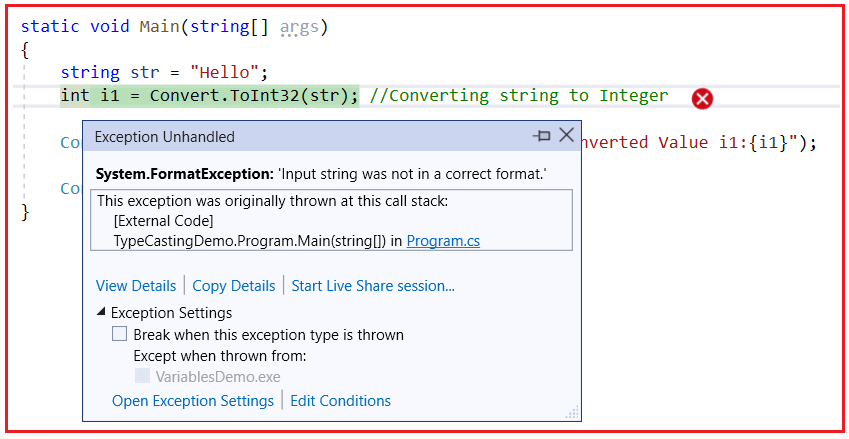##### Type Conversion using Parse() Method in C#

In C#, we can also use the built-in Parse() method to perform type conversion. So, while performing type conversion between non-compatible types like int and string, we can also use Parse() method like the Convert class helper methods. Now, if you go to the definition of built-in value data types such as int, short, long, bool, etc., then you will see that the Parse method is implemented as a static method in those built-in value data types. So, using the built-in type, we can call the Parse method.

For a better understanding, please have a look at the following example. In the below example, we are doing two incompatible type conversions. First, we are converting the string value 100 to integer type and in the second conversion, we are converting string to boolean.

```using System;
namespace TypeCastingDemo
{
class Program
{
static void Main(string[] args)
{
string str1 = "100";
//Converting string to int type
int i = int.Parse(str1);
Console.WriteLine(\$"Original String value: {str1} and Converted int value: {i}");

string str2 = "TRUE";
//Converting string to boolean type
bool b= bool.Parse(str2);
Console.WriteLine(\$"Original String value: {str2} and Converted bool value: {b}");
}
}
}
```

Now, when you run the above code, you will get the following output. Here, the values are compatible with type i.e. value 100 is compatible with int type, and value TRUE is compatible with bool type, and hence at runtime, these type conversions succeeded.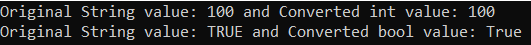Like the Convert class helper method, at runtime, if the value is not compatible with the destination type, then you will also get a runtime error. For a better understanding, please have a look at the below example where we are trying to store the string value Hello in the integer variable.

```using System;
namespace TypeCastingDemo
{
class Program
{
static void Main(string[] args)
{
string str1 = "Hello";
//Converting string to int type
int i = int.Parse(str1);
Console.WriteLine(\$"Original String value: {str1} and Converted int value: {i}");

}
}
}
```
###### Output: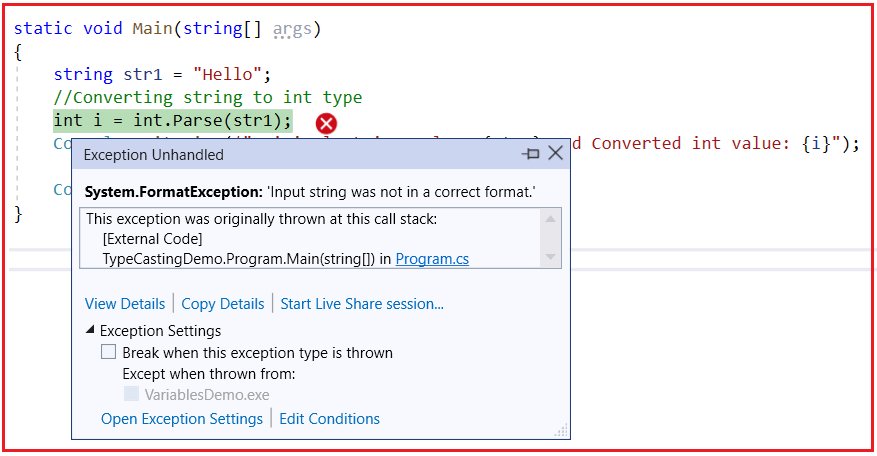##### Using TryParse Method in C#:

When we use the Parse method, if the conversion is not possible then at runtime, we will get an exception which is not a good thing. Because if conversion is not possible then we should show some information to the user and we should proceed further. To do so, the built-in value type class in C# is provided with the TryParse method. Let us see how to use the TryParse method in C#. Suppose, we want to convert a string to an integer type, then we can use the TryParse method as follows.

bool IsConverted = int.TryParse(“100”, out int I1);

Here, what the TryParse method will do is, it will try to convert the string value 100 to an integer type. If the conversion is successful, then it will do two things. First, it will store the converted value into the I1 variable and then it will return true. On the other hand, if the conversion is failed, then it will not store anything in the I1 variable and it will return false.

Let us understand this with an example. In the below example, the first conversion is successful and hence it will return true and will store the converted value 100 in the I1 variable. In the second conversion, the conversion failed and hence it will not store anything in the I2 variable and this time it will return false.

```using System;
namespace TypeCastingDemo
{
class Program
{
static void Main(string[] args)
{
string str1 = "100";
bool IsConverted1 = int.TryParse(str1, out int I1);
if (IsConverted1)
{
Console.WriteLine(\$"Original String value: {str1} and Converted int value: {I1}");
}
else
{
Console.WriteLine(\$"Try Parse Failed to Convert {str1} to integer");
}

string str2 = "Hello";
bool IsConverted2 = int.TryParse(str2, out int I2);
if (IsConverted2)
{
Console.WriteLine(\$"Original String value: {str2} and Converted int value: {I2}");
}
else
{
Console.WriteLine(\$"Try Parse Failed to Convert {str2} to integer");
}

}
}
}
```
###### Output: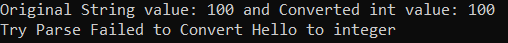1.2.3.